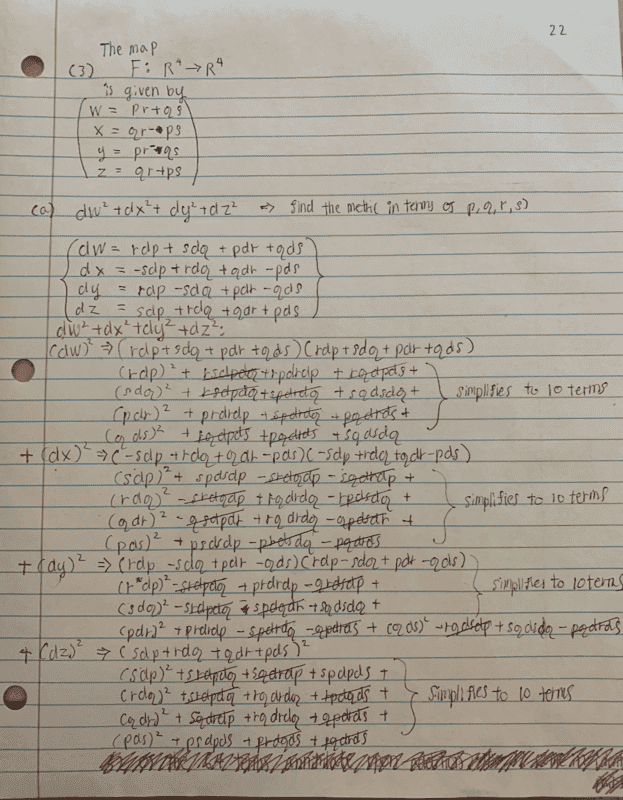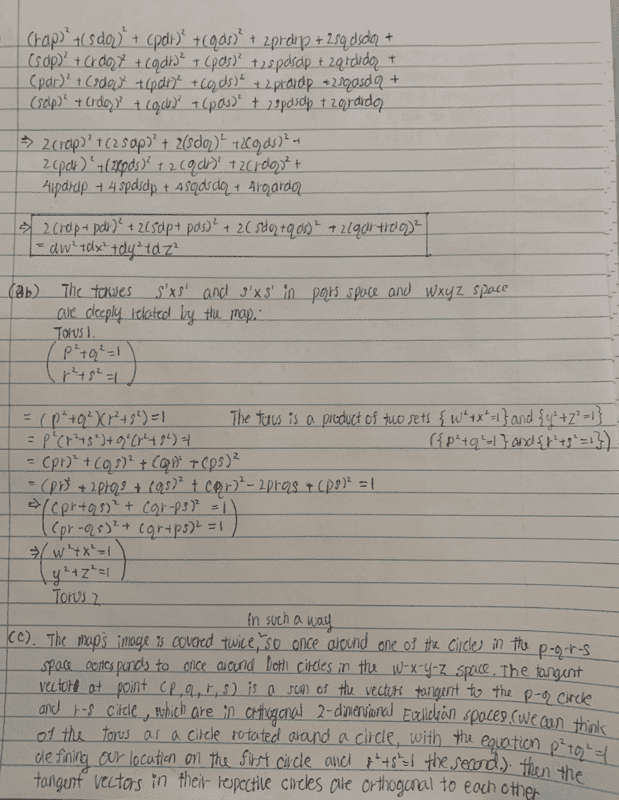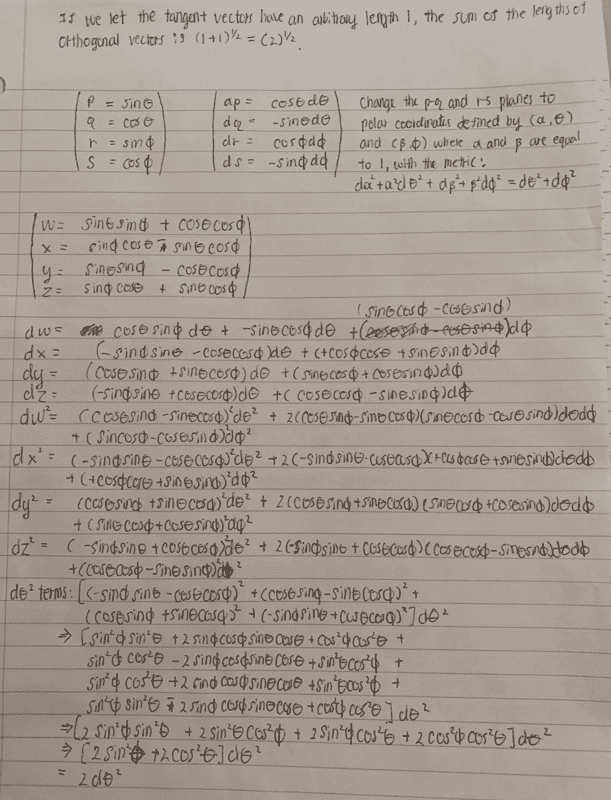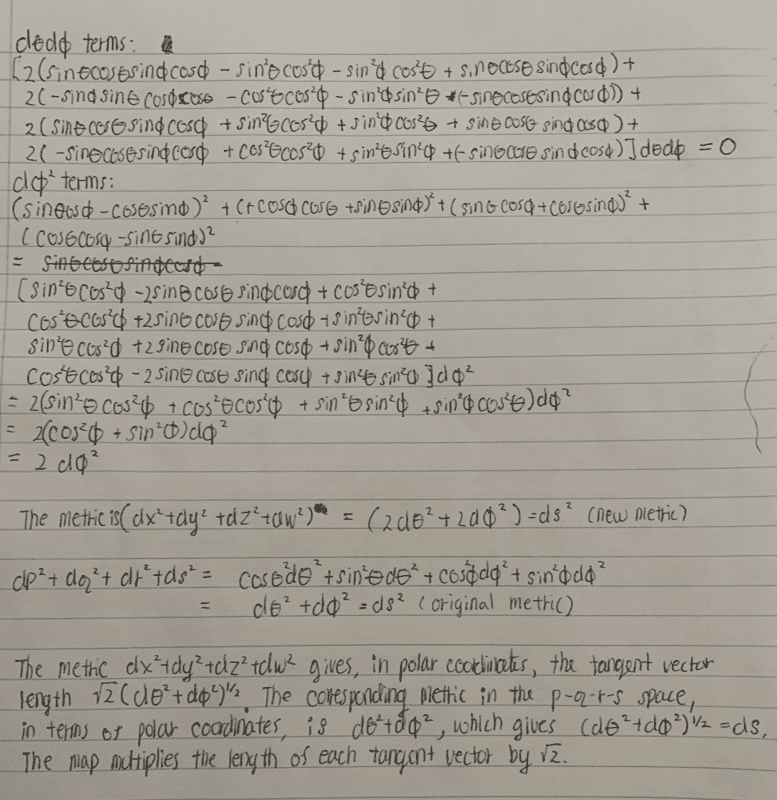# A multivariate function of Toruses - tangent vectors

docnet
Homework Statement:
this map multiplies all lengths of tangent vectors by the same factor. what is the factor?
Relevant Equations:
p^2+q^2=1
r^2+s^2=1

w^2+x^2=1
y^2+z^2=1

w=pr+qs
x=qr-ps
y=pr-qs
z=qr+ps
Thank you to all those who helped me solve my last question. This week, I've been assigned an interesting problem about toruses. I think I've solved most of this problem on my own, but I'd like to hear a few suggestions for part c.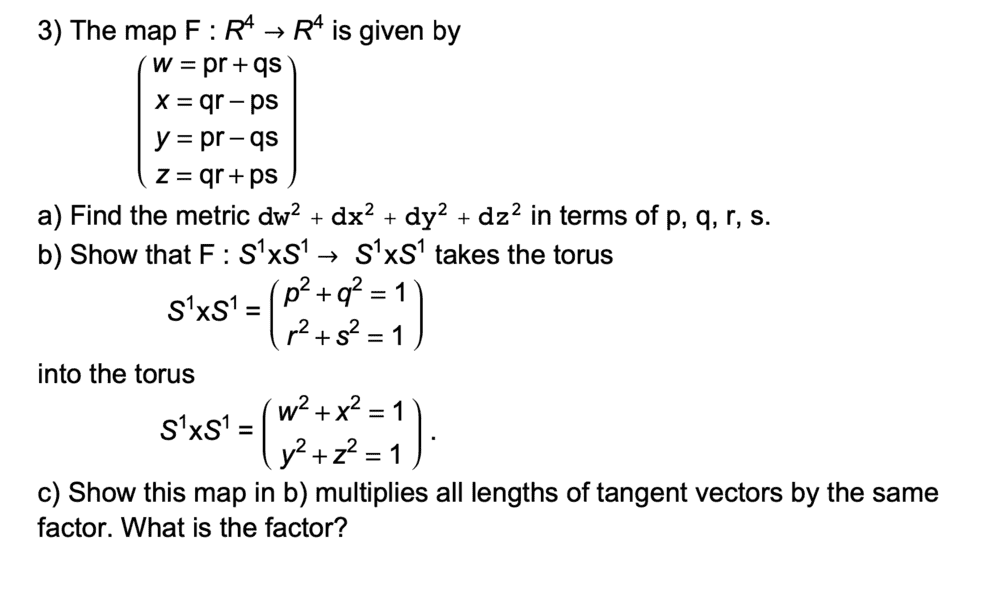I think this map multiplies tangent vectors by a factor of sqrt(2). We can think of tangent vectors as velocity while traveling along a curve on the surface. From simply plotting points, we know if travel around the pq plane or the rs plane once, we travel around both the wx and yz planes. We also know the two planes are orthogonal to each other because it's the definition of the torus under this equation. Under this map, the velocity gains an additional orthogonal component of equal magnitude, and from Pythagorean theorem the net magnitude is sqrt(1+1) = sqrt(2).

Is my intuition correct?

I've calculated the metric in part a and solved part b. but, I don't think these parts will help with c.

thanks

Staff Emeritus
Gold Member
The metric tells you how to compute the length of tangent vectors. Maybe it can help?

•docnet
Homework Helper
2022 Award
If you set $(p,q,r,s) = (\cos \theta, \sin \theta, \cos \phi, \sin \phi)$ you will find that in appropriate charts the map sends $(\theta, \phi)$ to $(\zeta, \eta) = (\theta - \phi, \theta + \phi)$. Thus a tangent vector $X = \alpha \frac{\partial}{\partial \theta} + \beta\frac{\partial}{\partial \phi}$ is mapped to $$Y = (\alpha + \beta)\frac{\partial}{\partial \zeta} + (\alpha - \beta)\frac{\partial}{\partial \eta}.$$ You can assume that all of the basis vectors are orthogonal with unit norm.

•docnet
docnet
The metric tells you how to compute the length of tangent vectors. Maybe it can help?

Thank you. I ended up using the metric and found the answer to be 2.

If you set (p,q,r,s)=(cos⁡θ,sin⁡θ,cos⁡ϕ,sin⁡ϕ) you will find that in appropriate charts the map sends (θ,ϕ) to (ζ,η)=(θ−ϕ,θ+ϕ). Thus a tangent vector X=α∂∂θ+β∂∂ϕ is mapped to Y=(α+β)∂∂ζ+(α−β)∂∂η. You can assume that all of the basis vectors are orthogonal with unit norm.

Sorry I'm having trouble visualizing these tangent vectors. How does one understand tangent vectors in polar coordinates?

Thanks,

#### Attachments

• Torus.pdf
824.4 KB · Views: 116
Last edited:
docnet
I realized my solutions are wrong. How do I delete my previous post?

docnet
Here are the complete solutions to the problem. Feel free to let me know if you see an error :)